# Why Do We Need To Minimize Influx (Kick)?

As you know, we’ve always been trained or told to minimize influx (kick). Nowadays, there are several tools and procedures guiding us to prevent large influx; however, interestingly there are quite a lot of people who don’t understand why we need to do this. In this topic, we will demonstrate how kick volume will affect wellbore and surface casing pressure.Main concept of minimizing kick coming into the wellbore is to minimize surface casing pressure when shut in. If you have excessive surface casing pressure, you will have a chance to fracture the weakest formation in the wellbore such as formation at casing shoe. You need to remember that more influx equals to more surface pressure. We will do basic calculation to see the effect of kick volume and surface pressure.

Example: Use the following information and compare the result of 2 cases.

Well Information (figure 1)Figure 1 – Well Information

• 9-5/8” casing shoe was set at 5,000’MD/5,000’TVD.
• The well is drilled to 10,000’MD/10,000’TVD with 8.5 bit.
• The well is assumed to be a gauge hole.
• Current mud weight is 9.2 ppg water based mud.
• Leak off test performed at 9-5/8” casing shoe is 13.5 ppg equivalent.
• Reservoir pressure at 10,000’ TVD is 10.5 ppg equivalent.
• Average gas gradient is 0.1 psi/ft.
• 5” DP is used to drill this section and 6-1/2” DC is used as BHA for 1,000 ft.

What will happen if the wellbore influx is 10 bbl and 50 bbl?

First of all, we need to determine influx height of 10 bbl and 50 bbl.

Influx Height = Kick Volume ÷ Annular Capacity

Annular Capacity between 8-1/2” hole and 5” DP = (8.52 – 52) ÷ 1029.4 = 0.04590 bbl/ft

Annular Capacity between 8-1/2” hole and 6.5” DC = (8.52 – 6.52) ÷ 1029.4 = 0.02194 bbl/ft

Height of 10 bbl

Influx Height @ 10 bbl = 10 ÷ 0.02194 = 343 ft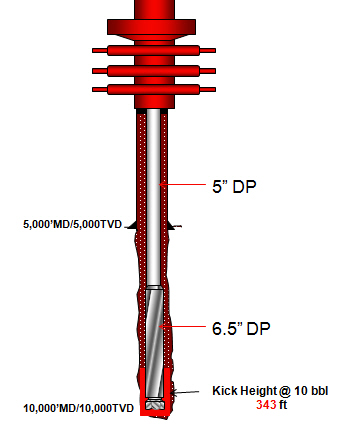Figure 2 – Height of 10 bbl kick

Height of 50 bbl

For this case, we need to check see if 50 bbl will be more than annular volume between hole and drill collar.

Volume between hole and 6.5” DC = Annular Capacity x DC Length

Volume between hole and 6.5” DC = 0.02914 x 1,000 = 29.14 bbl

As you can see from the figure, it tells us that there is kick volume in the annulus between hole and 5” DP.

Kick Volume between Hole and 6.5” DC = Total Kick Volume – Volume between hole and 6.5” DC

Kick Volume between Hole and 6.5” DC = 50 – 29.14 = 20.86 bbl

We know that we will have 20.86 bbl of kick between hole and 5” DP and then we need to calculate height of that volume.

Influx Height @ 20.86 bbl = 20.86 ÷ 0.04590 = 454 ft

Total Influx Height = Influx Height between DC and Hole + Influx Height between DP and Hole

Total Influx Height = 1000 + 454 = 1454 ft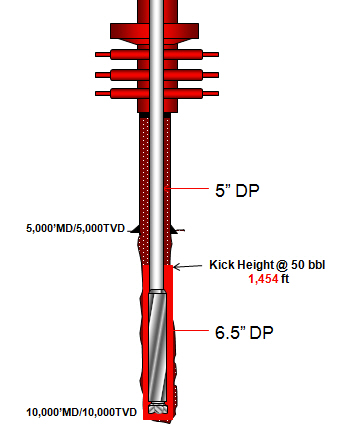Figure 3 – Height of 50 bbl kick

What is formation pressure at 10,000’MD/10,000TVD?

Formation pressure = 0.052 x 10.5 x 10,000 = 5,460 psi

What is Maximum Initial Shut-in Casing Pressure (MISICP)?

Maximum Initial Shut-in Casing Pressure (MISICP) = (LOT – Current MW) x 0.052 x Shoe TVD

Maximum Initial Shut-in Casing Pressure (MISICP) = (13.5 – 9.2) x 0.052 x 5,000 = 1,118 psi

Then we need to apply the hydrostatic pressure concept to determine casing pressure as per the relationship below.

Formation Pressure = Hydrostatic Pressure + Casing Pressure

Re-write to the equation below

Casing Pressure = Formation Pressure – Hydrostatic Pressure

Hydrostatic Pressure with 10 bbl of Kick in The Well

Hydrostatic Pressure = Hydrostatic from Gas + Hydrostatic from Mud

Hydrostatic Pressure = (Gas Gradient x Height of Gas) + (0.052 x MW X Height of Mud)

Hydrostatic Pressure = (0.1 x 343) + (0.052 x 9.2 x (10,000 – 343)) = 4,654 psi

Casing Pressure with 10 bbl of Kick in The Well

Casing Pressure = 5,460 – 4,654 = 806 psi (Figure 4)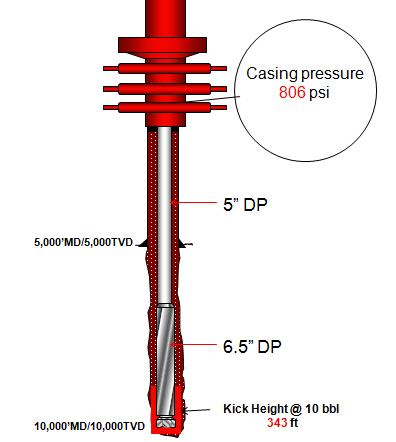Figure 4 – Casing Pressure with 10 bbl gas kick

Hydrostatic Pressure with 50 bbl of Kick in The Well

Hydrostatic Pressure = Hydrostatic from Gas + Hydrostatic from Mud

Hydrostatic Pressure = (Gas Gradient x Height of Gas) + (0.052 x MW X Height of Mud)

Hydrostatic Pressure = (0.1 x 1454) + (0.052 x 9.2 x (10,000 – 1545)) = 4,233 psi

Casing Pressure = 5,460 – 4,233 = 1,227 psi (Figure 5)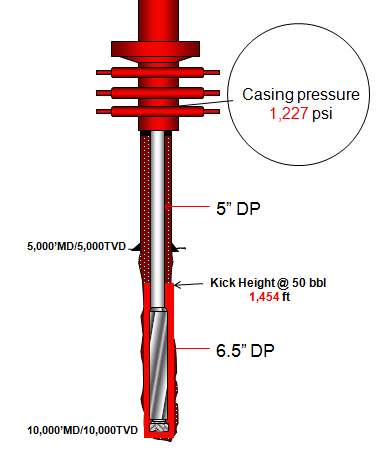Figure 5 – Casing Pressure with 50 bbl gas kick

Based on the same assumption, we will get the surface pressure as listed below

Casing Pressure with 10 bbl kick = 806 psi

Casing Pressure with 50 bbl kick = 1,227 psi

If we compare with MISICP of 1,118 psi from the calculation above, we will see that 50 bbl kick will break the casing shoe (Figure 6).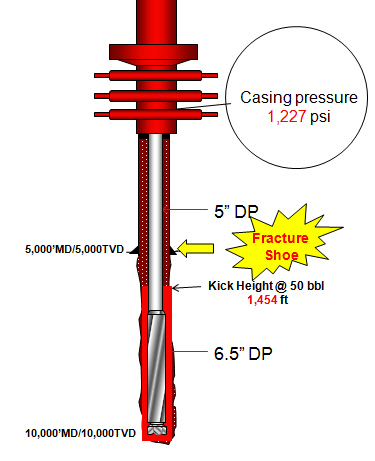Figure 6 – Shoe Fracture

### Conclusion

More Kick = More Surface Pressure = Less Safe

Less Kick = Less Surface Pressure = Safer

Reference book:Well Control Books

Share the joy
Tagged , , . Bookmark the permalink.1.Ali Javad says: